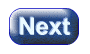created 07/18/2003

# CHAPTER 0 — Points and Lines

This is a tutorial on the mathematical tools used in Three Dimensional Computer Graphics (3D Graphics). A 3D graphics program builds a scene composed of three dimensional objects in three dimensional space. Then two dimensional images are produced from the 3D scene. The scene is represented as a data structure in computer memory. The 2D image is typically the picture on the face of a computer monitor.

The 3D objects are composed of points, lines, and polygons. You know what these are, but so that we can start from a common ground, this chapter reviews these concepts and introduces some of the vocabulary of computer graphics.

#### Chapter Topics:

• Points.
• Lines.
• Triangles.
• Vectors.
• Representing points, lines, and vectors.

The two fundamental objects in geometry are points and lines. What are they?

### QUESTION 1: